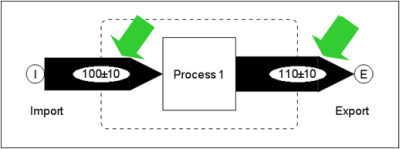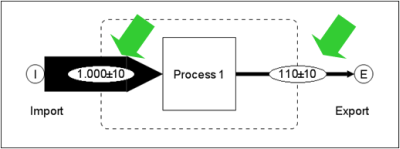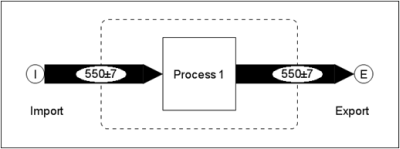Data Reconciliation

What is data reconciliation good for? Let´s look at a few examples. Starting point is the following model:In process 1 no buildup of a stock is allowed, thus following the law of mass conservation the import flow should equal the export flow. But that´s not the case i.e. there is a contradiction in given data.

These kinds of problems can be solved by data reconciliation. But there are two necessary conditions:

1. The system of equations has to be over determined (more independent equations than unknown variables).
2. Some of the given data have to be normally distributed.

During data reconciliation the mean values of uncertain data will be altered in a way that contradictions disappear. A solution is found when the sum of squares of the necessary chances reaches a minimum (method of least squares). The variances (square of standard deviation) of the uncertain quantities are used as weighing factors. As additional effect of data reconciliation the uncertainty of the reconciled data is reduced.

The example system can be described mathematically with one balance equation. Because there are no unknown variables present it is a over determined set of equations (more independent equations than unknown variables). In all following scenarios at least one of the two given flows has an uncertainty.

The first picture always shows the situation before and the following picture the situation after data reconciliation. Afterwards you find a short explanation.The export flow has been changed, because it is the only flow with an uncertainty.The mean values of import and export have been changed to the same extent (+/- 5) because both of them had the same absolute uncertainty given. Additional the uncertainties of the results have been reduced.The export flow has been changed stronger because it had the bigger absolute uncertainty.The mean values of import and export have been changed to the same extent because both of them had the same absolute uncertainty given. However, the import flow contained a gross error. One zero too much had been entered.

To find these kinds of errors STAN performs a statistical test to check if the necessary changes can be explained by random errors. You can think of it in the following way: if the reconciled value lies inside of the 95% confidence interval (mean value +/- 2* standard deviation) the data reconciliation will be accepted as correct. Otherwise it will be rejected an STAN displays a warning.A more complex model:The given values contain contradictions. For a closed balance of process 1 the flow "recycling material" should be 220 units, for a closed balance of process 2 210 units. This is a contradiction.

Data reconciliation can help again to solve the dilemma. Through transformation of the given set of equations (balance equations of process 1 and 2) one equation can be found that describes the balance equation of the subsystem displayed in green in the next picture.This equation contains no unknown variables (= over determined set of equations). Because some of the involved variables are uncertain they can be reconciled. The contradiction is resolved and the mean value of "recycling material" can be calculated. Its uncertainty is computed by error propagation.